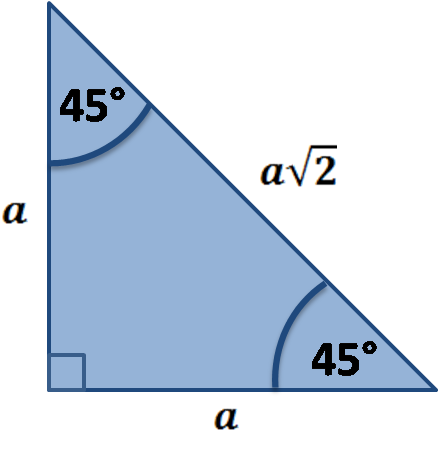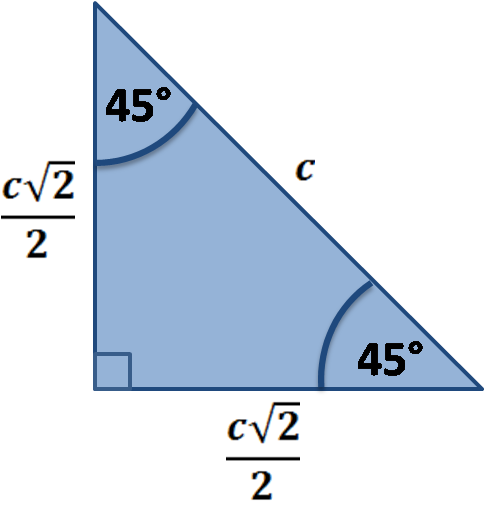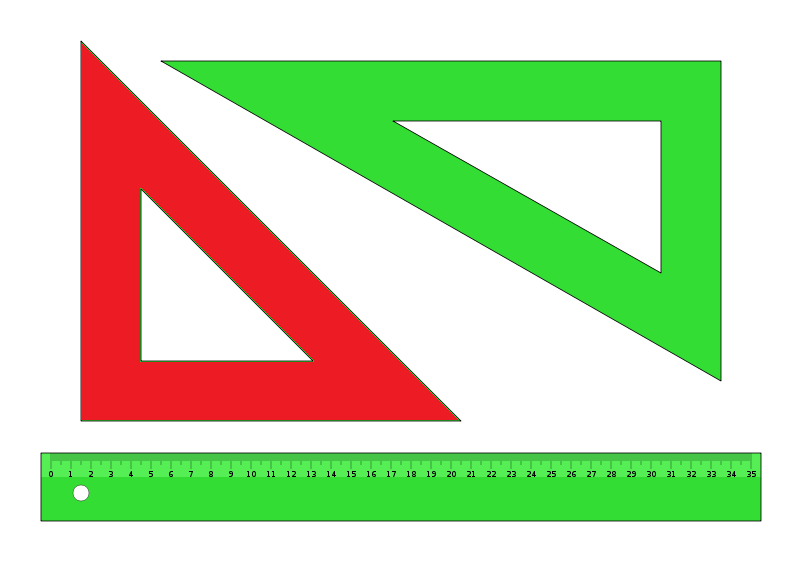# 45 45 90 Triangle Calculator

Created by Hanna Pamuła, PhD candidate
Reviewed by Bogna Szyk
Last updated: Nov 05, 2021

45 45 90 triangle calculator is a dedicated tool to solve this special right triangle. Find out what are the sides, hypotenuse, area and perimeter of your shape and learn about 45 45 90 triangle formula, ratio and rules.

If you want to know more about another popular right triangles, check out this 30 60 90 triangle tool and the calculator for special right triangles.

## How to solve a 45 45 90 triangle? 45 45 90 triangle formula

If you are wondering how to find the formula for 45 45 90 triangle hypotenuse, you're at a right place.
If the leg of the triangle is equal to a, then:

• the second leg is also equal to a
• the hypotenuse is a√2
• the area is equal to a²/2
• the perimeter equals a(2 + √2)

OK, looks easy, but where does it come from? There are a couple of methods to prove that equation, the most popular between them are:1. Using the Pythagorean theorem
• As you know one leg length a, you the know the length of the other as well, as both of them are equal.

• Find the hypotenuse from the Pythagorean theorem:

a² + b² = c² => a² + a² = c² so c = √(2a²) = a√2

1. Using the properties of the square

Did you notice that the 45 45 90 triangle is half of a square, cut along the square's diagonal?

• Again, we know that both legs are equal to a
• As you probably remember, the diagonal of the square is equal to side times square root of 2 - a√2. In our case, this diagonal is equal to the hypotenuse. That was quick!
1. Using trigonometry

If you heard about trigonometry, you could use the properties of sine and cosine. For this special angle of 45°, both of them are equal to √2/2. So:
a/c = √2/2 so c = a√2

To find the area of such triangle, use the basic triangle area formula is area = base * height / 2. In our case, one leg is a base and the other is the height, as there is a right angle between them. So the area of 45 45 90 triangles is:

area = a² / 2


To calculate the perimeter, simply add all 45 45 90 triangle sides:

perimeter = a + b + c = a + a + a√2 = a(2 + √2)

## 45 45 90 triangle sidesThe legs of such a triangle are equal, the hypotenuse is calculated immediately from the equation c = a√2. If the hypotenuse value is given, the side length will be equal to a = c√2/2.Triangles (set squares). The red one is the 45 45 90 degree angle triangle

## 45 45 90 triangle rules and properties

The most important rule is that this triangle has one right angle, and two other angles are equal to 45°. It implies that two sides - legs - are equal in length and the hypotenuse can be easily calculated. The other interesting properties of the 45 45 90 triangles are:

• It's the only possible right triangle that is also an isosceles triangle
• It has the smallest ratio of the hypotenuse to the sum of the legs
• It has the greatest ratio of the altitude from the hypotenuse to the sum of the legs

## 45 45 90 triangle ratioIn a 45 45 90 triangle, the ratios are equal to:

• 1 : 1 : 2 for angles (45° : 45° : 90°)
• 1 : 1 : √2 for sides (a : a : a√2)

## How to solve a 45 45 90 triangle: an example

Have a look at this real-life example to catch on the 45 45 90 triangle rules.

Assume we want to solve the isosceles triangle from a triangle set.

1. Type the given value. In our case, the easiest way is to type the length of the part with the scale. The usual leg length is 9 inches, so type that value into a or b box.
2. The 45 45 90 triangle calculator shows the remaining parameters. Now you know:
• hypotenuse length - 9 in * √2 = 12.73 in
• area - 9 in * 9 in / 2 = 40.5 in²
• perimeter - 9 in + 9 in + 9 in * √2 = 30.73 in

Remember that every time you can change the units displayed by simply clicking on the unit name. Also, don't forget that our calculator is a flexible tool - if you only know the area, the hypotenuse or even the perimeter, it can calculate the remaining parameters as well. Awesome!

Hanna Pamuła, PhD candidatea
in
b
in
c
in
Area
in²
Perimeter
in
People also viewed…

### Alien civilization

The alien civilization calculator explores the existence of extraterrestrial civilizations by comparing two models: the Drake equation and the Astrobiological Copernican Limits👽

### Meat footprint

Check out the meat impact - on the environment and your health.

### Pyramid volume

This pyramid volume calculator helps in fining the volume of a pyramids with a regular base or with known base area.

### Square in a circle

The square in a circle calculator is a perfect tool to find the biggest square that can fit in a circle. You can also use this calculator to find the largest circle that can fit inside a square, find the dimensions of a square with the same area as a circle, and find the dimensions of a circle with the same area as a square.